# design of ohmmeter

Though mechanical ohmmeter (resistance meter) designs are rarely used today, having largely been superseded by digital instruments, their operation is nonetheless intriguing and worthy of study.

The purpose of an ohmmeter, of course, is to measure the resistance placed between its leads. This resistance reading is indicated through a mechanical meter movement which operates on electric current. The ohmmeter must then have an internal source of voltage to create the necessary current to operate the movement, and also have appropriate ranging resistors to allow just the right amount of current through the movement at any given resistance.

Starting with a simple movement and battery circuit, let’s see how it would function as an ohmmeter: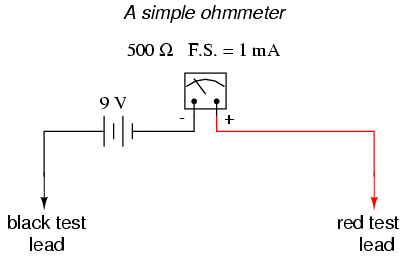When there is infinite resistance (no continuity between test leads), there is zero current through the meter movement, and the needle points toward the far left of the scale. In this regard, the ohmmeter indication is “backwards” because maximum indication (infinity) is on the left of the scale, while voltage and current meters have zero at the left of their scales.

If the test leads of this ohmmeter are directly shorted together (measuring zero Ω), the meter movement will have a maximum amount of current through it, limited only by the battery voltage and the movement’s internal resistance: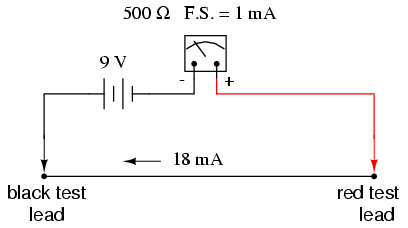With 9 volts of battery potential and only 500 Ω of movement resistance, our circuit current will be 18 mA, which is far beyond the full-scale rating of the movement. Such an excess of current will likely damage the meter.

Not only that, but having such a condition limits the usefulness of the device. If full left-of-scale on the meter face represents an infinite amount of resistance, then full right-of-scale should represent zero. Currently, our design “pegs” the meter movement hard to the right when zero resistance is attached between the leads. We need a way to make it so that the movement just registers full-scale when the test leads are shorted together. This is accomplished by adding a serie resistance to the meter’s circuit: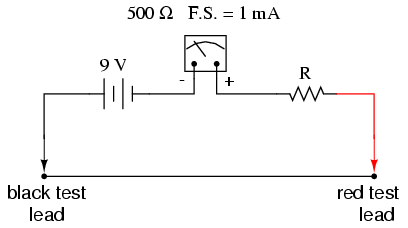To determine the proper value for R, we calculate the total circuit resistance needed to limit current to 1 mA (full-scale deflection on the movement) with 9 volts of potential from the battery, then subtract the movement’s internal resistance from that figure: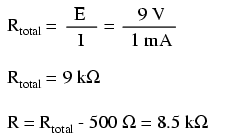Now that the right value for R has been calculated, we’re still left with a problem of meter range. On the left side of the scale we have “infinity” and on the right side we have zero. Besides being “backwards” from the scales of voltmeters and ammeters, this scale is strange because it goes from nothing to everything, rather than from nothing to a finite value (such as 10 volts, 1 amp, etc.). One might pause to wonder, “what does middle-of-scale represent? What figure lies exactly between zero and infinity?” Infinity is more than just a very big amount: it is an incalculable quantity, larger than any definite number ever could be. If half-scale indication on any other type of meter represents 1/2 of the full-scale range value, then what is half of infinity on an ohmmeter scale?

The answer to this paradox is a logarithmic scale. Simply put, the scale of an ohmmeter does not smoothly progress from zero to infinity as the needle sweeps from right to left. Rather, the scale starts out “expanded” at the right-hand side, with the successive resistance values growing closer and closer to each other toward the left side of the scale: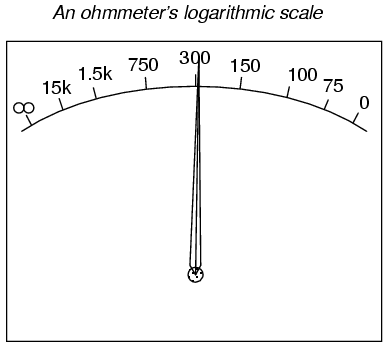Infinity cannot be approached in a linear (even) fashion, because the scale would never get there! With a logarithmic scale, the amount of resistance spanned for any given distance on the scale increases as the scale progresses toward infinity, making infinity an attainable goal.

We still have a question of range for our ohmmeter, though. What value of resistance between the test leads will cause exactly 1/2 scale deflection of the needle? If we know that the movement has a full-scale rating of 1 mA, then 0.5 mA (500 µA) must be the value needed for half-scale deflection. Following our design with the 9 volt battery as a source we get:With an internal movement resistance of 500 Ω and a series range resistor of 8.5 kΩ, this leaves 9 kΩ for an external (lead-to-lead) test resistance at 1/2 scale. In other words, the test resistance giving 1/2 scale deflection in an ohmmeter is equal in value to the (internal) series total resistance of the meter circuit.

Using Ohm’s Law a few more times, we can determine the test resistance value for 1/4 and 3/4 scale deflection as well:

1/4 scale deflection (0.25 mA of meter current):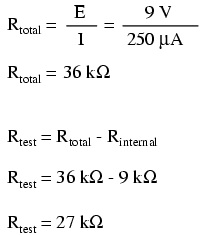3/4 scale deflection (0.75 mA of meter current):So, the scale for this ohmmeter looks something like this:One major problem with this design is its reliance upon a stable battery voltage for accurate resistance reading. If the battery voltage decreases (as all chemical batteries do with age and use), the ohmmeter scale will lose accuracy. With the series range resistor at a constant value of 8.5 kΩ and the battery voltage decreasing, the meter will no longer deflect full-scale to the right when the test leads are shorted together (0 Ω). Likewise, a test resistance of 9 kΩ will fail to deflect the needle to exactly 1/2 scale with a lesser battery voltage.

There are design techniques used to compensate for varying battery voltage, but they do not completely take care of the problem and are to be considered approximations at best. For this reason, and for the fact of the logarithmic scale, this type of ohmmeter is never considered to be a precision instrument.

One final caveat needs to be mentioned with regard to ohmmeers: they only function correctly when measuring resistance that is not being powered by a voltage or current source. In other words, you cannot measure resistance with an ohmmeter on a “live” circuit! The reason for this is simple: the ohmmeter’s accurate indication depends on the only source of voltage being its internal battery. The presence of any voltage across the component to be measured will interfere with the ohmmeter’s operation. If the voltage is large enough, it may even damage the ohmmeter.

REVIEW:
Ohmmeters contain internal sources of voltage to supply power in taking resistance measurements.
An analog ohmmeter scale is “backwards” from that of a voltmeter or ammeter, the movement needle reading zero resistance at full-scale and infinite resistance at rest.
Analog ohmmeters also have logarithmic scales, “expanded” at the low end of the scale and “compressed” at the high end to be able to span from zero to infinite resistance.
Analog ohmmeters are not precision instruments.
Ohmmeters should never be connected to an energized circuit (that is, a circuit with its own source of voltage). Any voltage applied to the test leads of an ohmmeter will invalidate its reading.

## High voltage ohmmeters

Most ohmmeters of the design shown in the previous section utilize a battery of relatively low voltage, usually nine volts or less. This is perfectly adequate for measuring resistances under several mega-ohms (MΩ), but when extremely high resistances need to be measured, a 9 volt battery is insufficient for generating enough current to actuate an electromechanical meter movement.

Also, as discussed in an earlier chapter, resistance is not always a stable (linear) quantity. This is especially true of non-metals. Recall the graph of current over voltage for a small air gap (less than an inch):While this is an extreme example of nonlinear conduction, other substances exhibit similar insulating/conducting properties when exposed to high voltages. Obviously, an ohmmeter using a low-voltage battery as a source of power cannot measure resistance at the ionization potential of a gas, or at the breakdown voltage of an insulator. If such resistance values need to be measured, nothing but a high-voltage ohmmeter will suffice.

The most direct method of high-voltage resistance measurement involves simply substituting a higher voltage battery in the same basic design of ohmmeter investigated earlier: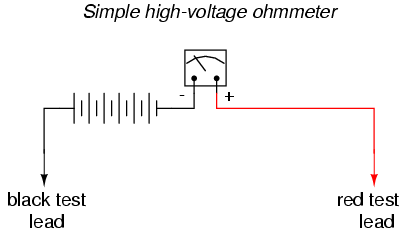Knowing, however, that the resistance of some materials tends to change with applied voltage, it would be advantageous to be able to adjust the voltage of this ohmmeter to obtain resistance measurements under different conditions: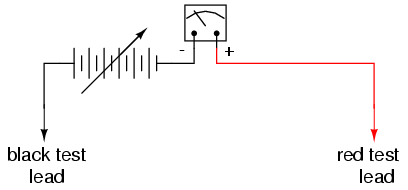Unfortunately, this would create a calibration problem for the meter. If the meter movement deflects full-scale with a certain amount of current through it, the full-scale range of the meter in ohms would change as the source voltage changed. Imagine connecting a stable resistance across the test leads of this ohmmeter while varying the source voltage: as the voltage is increased, there will be more current through the meter movement, hence a greater amount of deflection. What we really need is a meter movement that will produce a consistent, stable deflection for any stable resistance value measured, regardless of the applied voltage.

Accomplishing this design goal requires a special meter movement, one that is peculiar to megohmmeters, or meggers, as these instruments are known.The numbered, rectangular blocks in the above illustration are cross-sectional representations of wire coils. These three coils all move with the needle mechanism. There is no spring mechanism to return the needle to a set position. When the movement is unpowered, the needle will randomly “float.” The coils are electrically connected ike this:With infinite resistance between the test leads (open circuit), there will be no current through coil 1, only through coils 2 and 3. When energized, these coils try to center themselves in the gap between the two magnet poles, driving the needle fully to the right of the scale where it points to “infinity.”Any current through coil 1 (through a measured resistance connected between the test leads) tends to drive the needle to the left of scale, back to zero. The internal resistor values of the meter movement are calibrated so that when the test leads are shorted together, the needle deflects exactly to the 0 Ω position.

Because any variations in battery voltage will affect the torque generated by both sets of coils (coils 2 and 3, which drive the needle to the right, and coil 1, which drives the needle to the left), those variations will have no effect of the calibration of the movement. In other words, the accuracy of this ohmmeter movement is unaffected by battery voltage: a given amount of measured resistance will produce a certain needle deflection, no matter how much or little battery voltage is present.

The only effect that a variation in voltage will have on meter indication is the degree to which the measured resistance changes with applied voltage. So, if we were to use a megger to measure the resistance of a gas-discharge lamp, it would read very high resistance (needle to the far right of the scale) for low voltages and low resistance (needle moves to the left of the scale) for high voltages. This is precisely what we expect from a good high-voltage ohmmeter: to provide accurate indication of subject resistance under different circumstances.

For maximum safety, most meggers are equipped with hand-crank generators for producing the high DC voltage (up to 1000 volts). If the operator of the meter receives a shock from the high voltage, the condition will be self-correcting, as he or she will naturally stop cranking the generator! Sometimes a “slip clutch” is used to stabilize generator speed under different cranking conditions, so as to provide a fairly stable voltage whether it is cranked fast or slow. Multiple voltage output levels from the generator are available by the setting of a selector switch.

A simple hand-crank megger is shown in this photograph:width=”480″ height=”359″/>

Some meggers are battery-powered to provide greater precision in output voltage. For safety reasons these meggers are activated by a momentary-contact pushbutton switch, so the switch cannot be left in the “on” position and pose a significant shock hazard to the meter operator.

Real meggers are equipped with three connection terminals, labeled Line, Earth, and Guard. The schematic is quite similar to the simplified version shown earlier:Resistance is measured between the Line and Earth terminals, where current will travel through coil 1. The “Guard” terminal is provided for special testing situations where one resistance must be isolated from another. Take for instance this scenario where the insulation resistance is to be tested in a two-wire cable: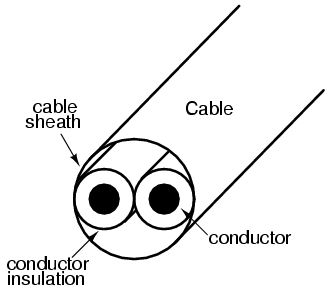To measure insulation resistance from a conductor to the outside of the cable, we need to connect the “Line” lead of the megger to one of the conductors and connect the “Earth” lead of the megger to a wire wrapped around the sheath of the cable:In this configuration the megger should read the resistance between one conductor and the outside sheath. Or will it? If we draw a schematic diagram showing all insulation resistances as resistor symbols, what we have looks like this:Rather than just measure the resistance of the second conductor to the sheath (Rc2-s), wha we’ll actually measure is that resistance in parallel with the series combination of conductor-to-conductor resistance (Rc1-c2) and the first conductor to the sheath (Rc1-s). If we don’t care about this fact, we can proceed with the test as configured. If we desire to measure only the resistance between the second conductor and the sheath (Rc2-s), then we need to use the megger’s “Guard” terminal:Now the circuit schematic looks like this: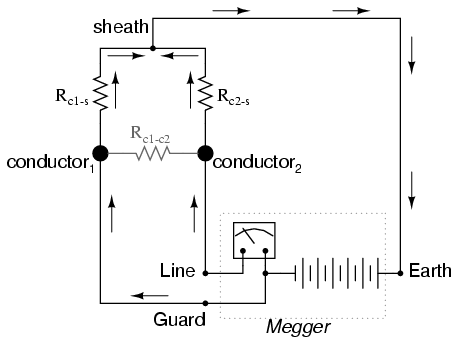Connecting the “Guard” terminal to the first conductor places the two conductors at almost equal potential. With little or no voltage between them, the insulation resistance is nearly infinite, and thus there will be no current between the two conductors. Consequently, the megger’s resistance indication will be based exclusively on the current through the second conductor’s insulation, through the cable sheath, and to the wire wrapped around, not the current leaking through the first conductor’s insulation.

Meggers are field instruments: that is, they are designed to be portable and operated by a technician on the job site with as much ease as a regular ohmmeter. They are very useful for checking high-resistance “short” failures between wires caused by wet or degraded insulation. Because they utilize such high voltages, they are not as affected by stray voltages (voltages less than 1 volt produced by electrochemical reactions between conductors, or “induced” by neighboring magnetic fields) as ordinary ohmmeters.

For a more thorough test of wire insulation, another high-voltage ohmmeter commonly called a hi-pot tester is used. These specialized instruments produce voltages in excess of 1 kV, and may be used for testing the insulating effectiveness of oil, ceramic insulators, and even the integrity of other high-voltage instruments. Because they are capable of producing such high voltages, they must be operated with the utmost care, and only by trained personnel.

It should be noted that hi-pot testers and even meggers (in certain conditions) are capable of damaging wire insulation if incorrectly used. Once an insulating material has been subjected to breakdown by the application of an excessive voltage, its ability to electrically insulate will be compromised. Again, these instruments are to be used only by trained personnel.

Related

COMMENT songs

1. som dutt

why ohms starts from infinity?